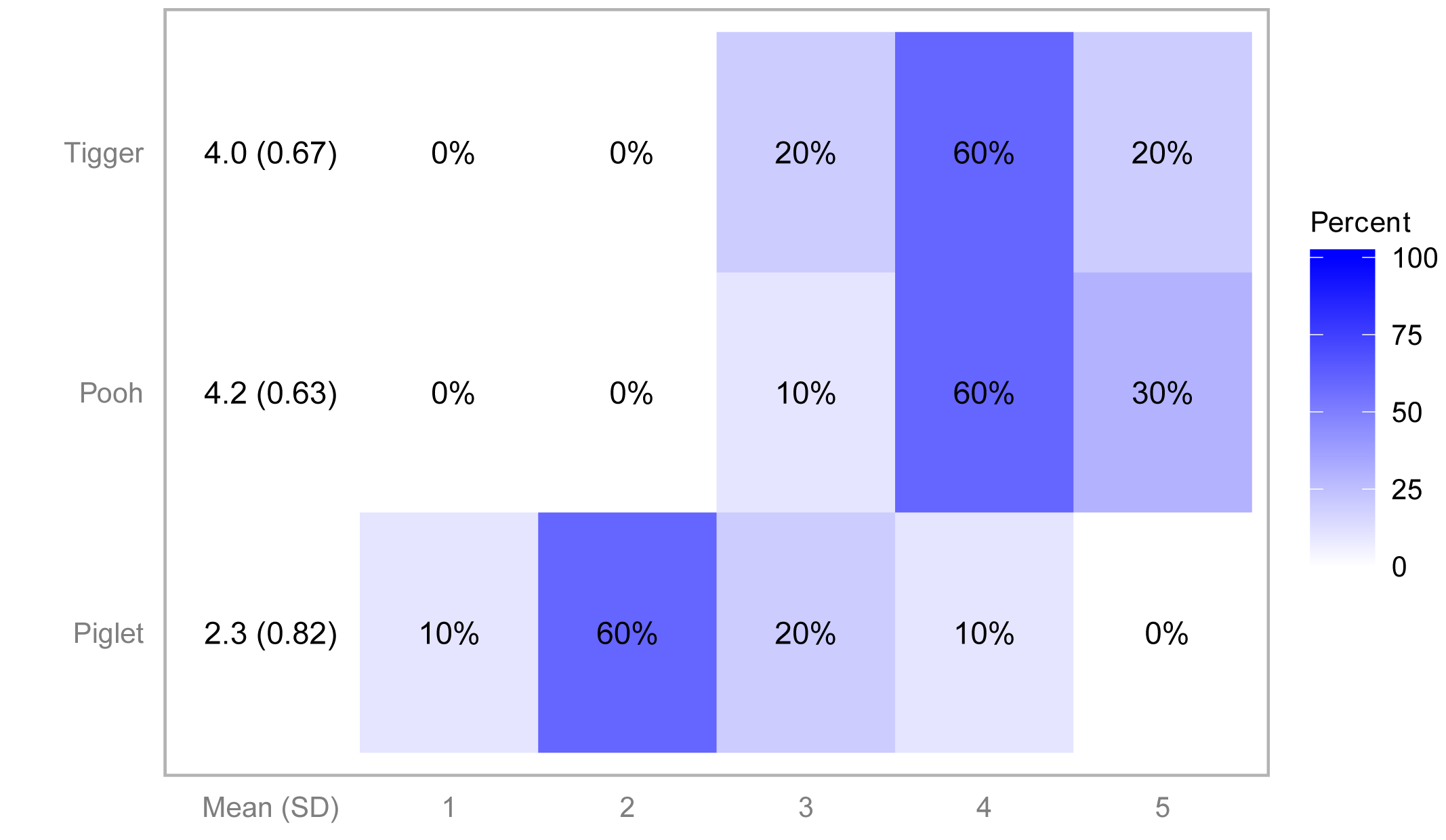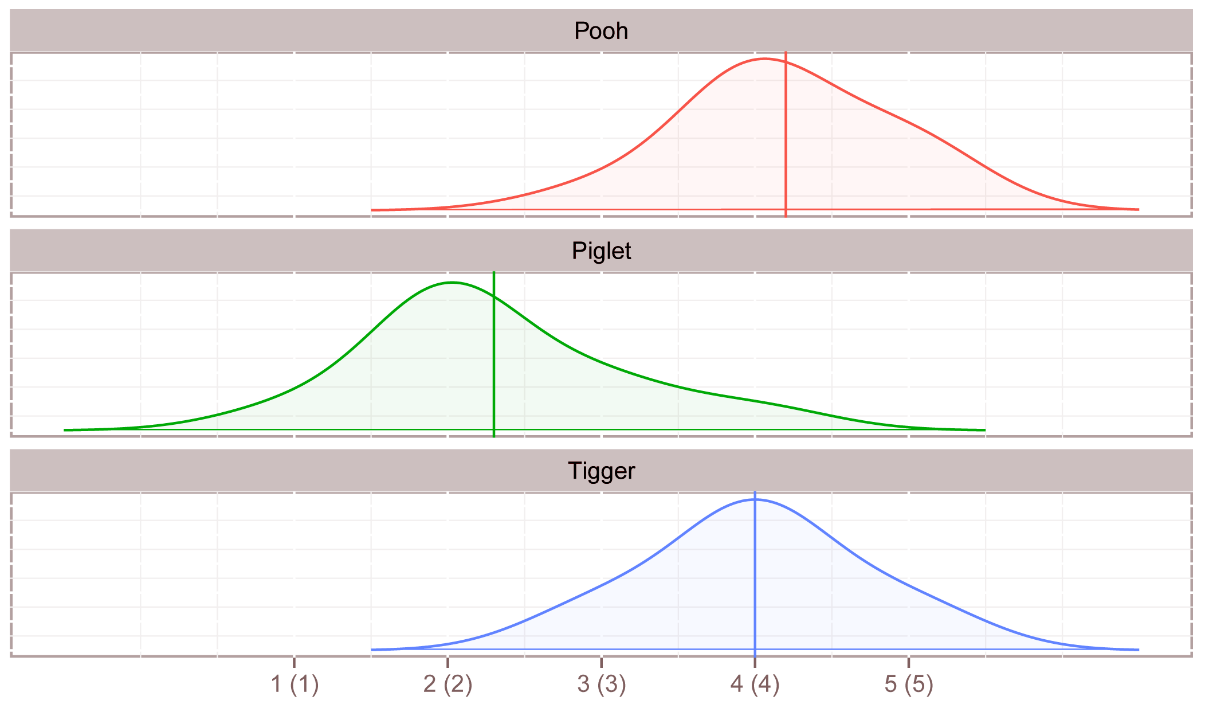## Summary and Analysis of Extension Program Evaluation in R

Salvatore S. Mangiafico

# Descriptive Statistics for Likert Item Data

The likert package can be used to produce attractive summaries and plots of one-sample or one-way Likert data.  The package is somewhat finicky with the form the data it accepts, however.  Data must be in “wide” format.  Long-format and wide-format data are discussed in the section “Long-format and wide-format data” in the Types of Variables chapter.  In addition, sample sizes for each level of the factor variable must be equal, but you can use NA values for missing observations.

### Packages used in this chapter

The packages used in this chapter include:

•  psych

•  likert

The following commands will install these packages if they are not already installed:

if(!require(psych)){install.packages("psych")}
if(!require(likert)){install.packages("likert")}

### The likert package

Input =("
Pooh    Piglet  Tigger
3       2       4
5       4       4
4       2       4
4       2       4
4       1       5
4       2       3
4       3       5
4       2       4
5       2       4
5       3       3
")

### Change Likert scores to factor and specify levels

Data\$Pooh = factor(Data\$Pooh,
levels = c("1", "2", "3", "4", "5"),
ordered = TRUE)

Data\$Piglet = factor(Data\$Piglet,
levels = c("1", "2", "3", "4", "5"),
ordered = TRUE)

Data\$Tigger = factor(Data\$Tigger,
levels = c("1", "2", "3", "4", "5"),
ordered = TRUE)

### Double check the data frame

library(psych)

str(Data)

'data.frame':     10 obs. of  3 variables:
\$ Pooh  : Ord.factor w/ 5 levels "1"<"2"<"3"<"4"<..: 3 5 4 4 4 4 4 4 5 5
\$ Piglet: Ord.factor w/ 5 levels "1"<"2"<"3"<"4"<..: 2 4 2 2 1 2 3 2 2 3
\$ Tigger: Ord.factor w/ 5 levels "1"<"2"<"3"<"4"<..: 4 4 4 4 5 3 5 4 4 3

summary(Data)

### Remove unnecessary objects

rm(Input)

### Summary statistics and plots with the likert package

##### Percent responses in each group

library(likert)

likert(Data)

Item  1  2  3  4  5
1   Pooh  0  0 10 60 30
2 Piglet 10 60 20 10  0
3 Tigger  0  0 20 60 20

### Note: if there are NA’s, this summary doesn’t tell you!

### Count responses with summary function in native stats package

summary(Data)

Pooh  Piglet Tigger
1:0   1:1    1:0
2:0   2:6    2:0
3:1   3:2    3:2
4:6   4:1    4:6
5:3   5:0    5:2

### Note: if there are NA’s, this summary will tell you

library(likert)

Result = likert(Data)

summary(Result)

Item low neutral high mean        sd
1   Pooh   0      10   90  4.2 0.6324555
3 Tigger   0      20   80  4.0 0.6666667
2 Piglet  70      20   10  2.3 0.8232726

### Note: responses are grouped into "low", "neutral", and "high"

### Note: if there are NA’s, this summary doesn’t tell you!

#### Plots that treat Likert data like factor data

##### Bar plot

library(likert)

Result = likert(Data)

plot(Result,
type="bar")### Note: for the percent numbers,
###   responses are grouped into "low", "neutral", and "high"

##### Heat plot

library(likert)

Result = likert(Data)

plot(Result,
type="heat",
low.color = "white",
high.color = "blue",
text.color = "black",
text.size = 4,
wrap = 50)#### Plots that treat Likert data like numeric data

##### Density plot

library(likert)

Result = likert(Data)

plot(Result,
type="density",
facet = TRUE,
bw = 0.5)### Note: Vertical lines are means for each group.

### Note: Curves are density plots, which show the distribution of values
###   similar to a histogram.

### References

Jason Bryer, J. and Speerschneider, K. likert: An R package analyzing and visualizing Likert items.

Jason Bryer, J. and Speerschneider, K. Package ‘likert’. cran.r-project.org/web/packages/likert/likert.pdf.